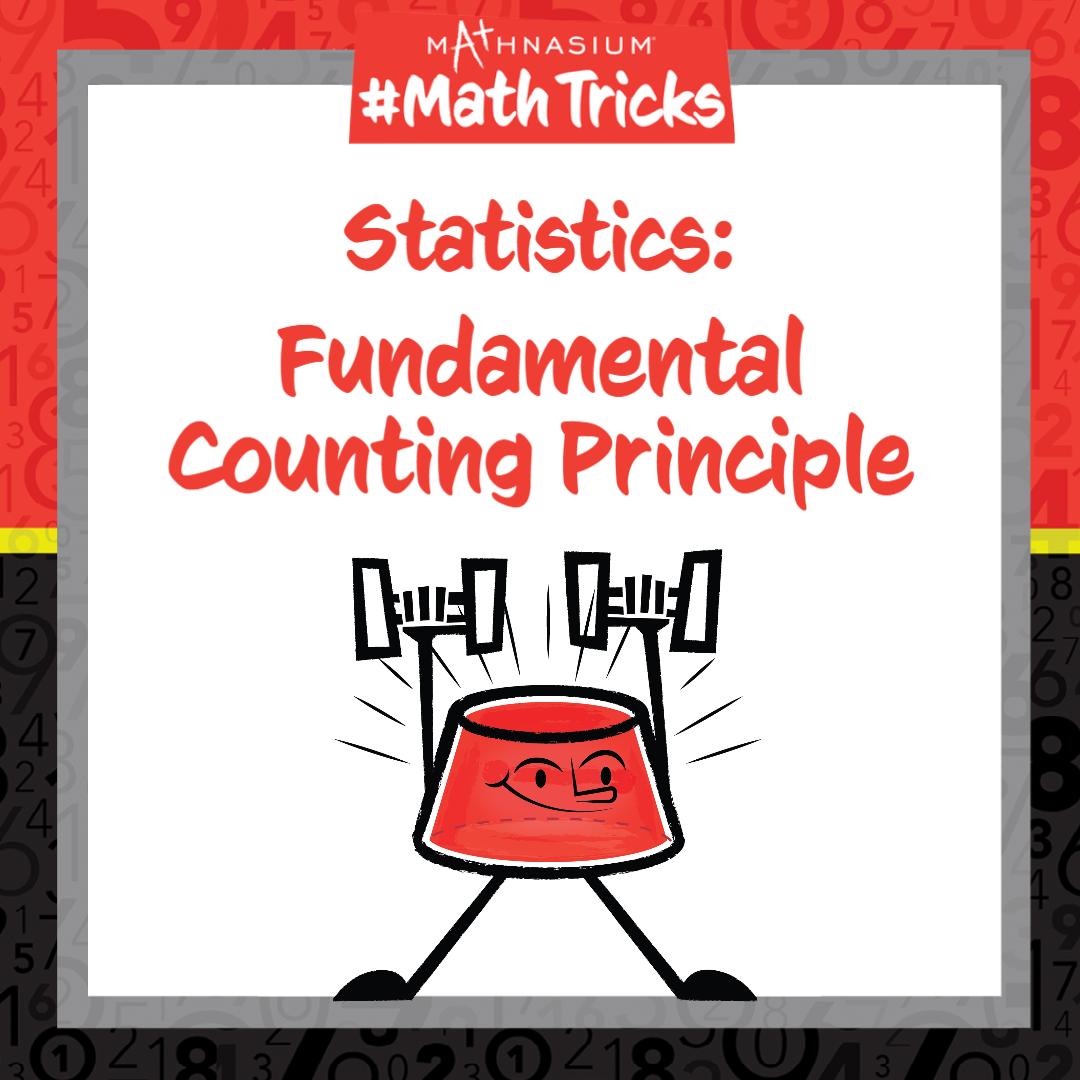877-601-6284
Get Started by Finding a Local Center

# Mathnasium #MathTricks: Statistics (Counting Part 1)

Apr 20, 2022Welcome to Mathnasium’s Math Tricks series. Whether it is in statistics or the real world, we are occasionally required to count large amounts. It can be a long and tedious process to list the total number of combinations for given events. So today we are showing you how to use the Fundamental Counting Principle to count the number of outcomes; the trick is to multiply the number of possibilities for each event together.

Use the Fundamental Counting Principle to find the number of combinations in the example below.

##### Example: How many 3-digit numbers satisfy all of the following conditions?

The first digit is greater than 6.
The second digit is an odd number.
The number is divisible by 2.

Step 1: Identify the possibilities for each condition.
The first digit can be: 7, 8, 9.
The second digit can be: 1, 3, 5, 7, 9.
The 3-digit number must end in an even number to be divisible by two: 0, 2, 4, 6, 8.

Step 2: Identify the number of possibilities for each condition.
The first digit has 3 possibilities.
The second digit has 5 possibilities.
The third digit has 5 possibilities.

Step 3: Multiply the number of possibilities for each condition.
3 x 5 x 5 = 75

Now, with the Fundamental Counting Principle, you are ready to solve counting problems. Click here for more practice problems, then check your answers here.

If you missed this or any of our other Math Tricks videos, check them out on our YouTube channel!

## SEE HOW MATHNASIUM WORKS FOR YOUR SITUATION

### My Child is:## OUR METHOD WORKS

Mathnasium meets your child where they are and helps them with the customized program they need, for any level of mathematics.Early LearnersElementary SchoolMiddle SchoolHigh School